Work, Power And Energy MCQ Level - 1

# Work, Power And Energy MCQ Level - 1

Test Description

## 10 Questions MCQ Test Topic wise Tests for IIT JAM Physics | Work, Power And Energy MCQ Level - 1

Work, Power And Energy MCQ Level - 1 for IIT JAM 2023 is part of Topic wise Tests for IIT JAM Physics preparation. The Work, Power And Energy MCQ Level - 1 questions and answers have been prepared according to the IIT JAM exam syllabus.The Work, Power And Energy MCQ Level - 1 MCQs are made for IIT JAM 2023 Exam. Find important definitions, questions, notes, meanings, examples, exercises, MCQs and online tests for Work, Power And Energy MCQ Level - 1 below.
Solutions of Work, Power And Energy MCQ Level - 1 questions in English are available as part of our Topic wise Tests for IIT JAM Physics for IIT JAM & Work, Power And Energy MCQ Level - 1 solutions in Hindi for Topic wise Tests for IIT JAM Physics course. Download more important topics, notes, lectures and mock test series for IIT JAM Exam by signing up for free. Attempt Work, Power And Energy MCQ Level - 1 | 10 questions in 30 minutes | Mock test for IIT JAM preparation | Free important questions MCQ to study Topic wise Tests for IIT JAM Physics for IIT JAM Exam | Download free PDF with solutions
 1 Crore+ students have signed up on EduRev. Have you?
Work, Power And Energy MCQ Level - 1 - Question 1

### A ring of mass m can slide over a smooth vertical rod. The ring is connected to a spring of force constant k = 4mg/R  where 2R is the natural length of the spring. The other end of the spring is fixed to the ground at a horizontal distance 2R  from the base of the rod. The mass is released at a height of 1.5R from the ground.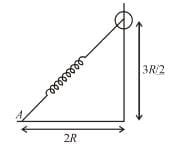Detailed Solution for Work, Power And Energy MCQ Level - 1 - Question 1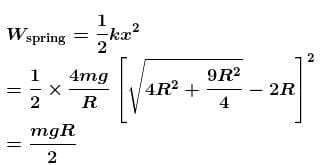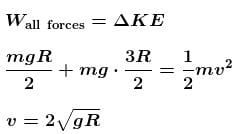The correct answer is: the velocity of the ring when it reaches the ground will be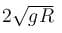Work, Power And Energy MCQ Level - 1 - Question 2

### A body is dropped from a certain height. When it loses U amount of its energy it acquires a velocity v. The mass of the body is :

Detailed Solution for Work, Power And Energy MCQ Level - 1 - Question 2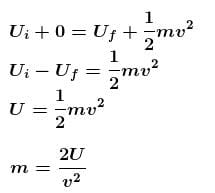The correct answer is: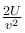Work, Power And Energy MCQ Level - 1 - Question 3

### A body is moved along a straight line by a machine delivering a constant power. The distance moved by the body in time t is proportional to :

Detailed Solution for Work, Power And Energy MCQ Level - 1 - Question 3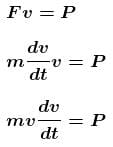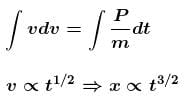Work, Power And Energy MCQ Level - 1 - Question 4

A self propelled vehicle of mass m whose engine delivers constant power P has an acceleration a = P/mv (assume that there is no friction). In order to increase its velocity from v1 to v2 the distance it has to travel will be :

Detailed Solution for Work, Power And Energy MCQ Level - 1 - Question 4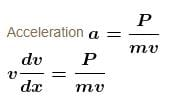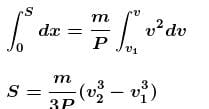The correct answer is: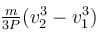Work, Power And Energy MCQ Level - 1 - Question 5

A block attached to a spring, pulled by a constant horizontal force, is kept on a smooth surface as shown in the figure. Initially, the spring is in the natural state. Then the maximum positive work that the applied force F can do is :

[Given that spring does not break]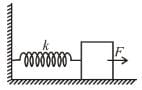Detailed Solution for Work, Power And Energy MCQ Level - 1 - Question 5

Applying work-energy theorem on the block, we get
Fl − kt2/2 = 0
l = 2F/k
or work done = Fl = 2F2/k

Work, Power And Energy MCQ Level - 1 - Question 6

A block of mass m is attached to two unstretched springs of spring constants k1 and k2 as shown in figure. The block is displaced towards right through a distance x and is released. Find the speed of the block as passes through the means position shown.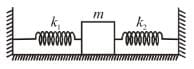Detailed Solution for Work, Power And Energy MCQ Level - 1 - Question 6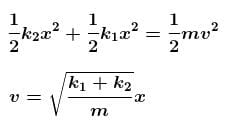The correct answer is: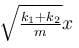Work, Power And Energy MCQ Level - 1 - Question 7

The block of mass m initially at x = 0 is acted upon by a horizontal force at any position x is given as F = a  bx2, where a > μmg, as shown in the figure. The co-efficient of friction between the surfaces of contact is μ. The net work done on the blocks is zero. If the block travels a distance?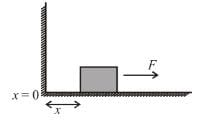Detailed Solution for Work, Power And Energy MCQ Level - 1 - Question 7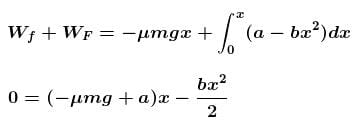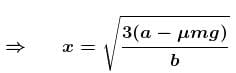The correct answer is: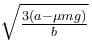Work, Power And Energy MCQ Level - 1 - Question 8

A man places a chain (of mass m and length l) on a table slowly. Initially the lower end of the chain just touches the table. The man drops the chain. When half of the chain is in vertical position. The work done by the man in this process is :

Detailed Solution for Work, Power And Energy MCQ Level - 1 - Question 8

The work done by man is negative of magnitude of decrease in potential energy of chain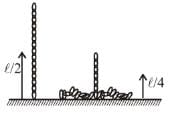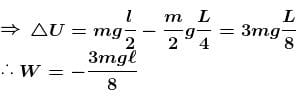The correct answer is: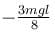Work, Power And Energy MCQ Level - 1 - Question 9

A body is projected with kinetic energy k at angle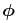with the vertical. Neglecting friction, its potential energy at the highest point will be :

Detailed Solution for Work, Power And Energy MCQ Level - 1 - Question 9

KE + PE = k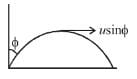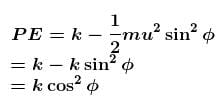The correct answer is: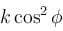Work, Power And Energy MCQ Level - 1 - Question 10

In the Figure, the ball A is released from rest when the spring is at its natural length. For the block B of mass M to leave contact with the ground of some stage, the minimum mass of A must be :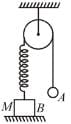Detailed Solution for Work, Power And Energy MCQ Level - 1 - Question 10

Let m be minimum mass of ball
Let mass A moves downwards by x.
From conservation of energy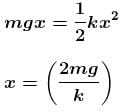For mass  to leave contact with ground,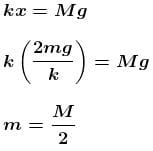## Topic wise Tests for IIT JAM Physics

217 tests
 Use Code STAYHOME200 and get INR 200 additional OFF Use Coupon Code
Information about Work, Power And Energy MCQ Level - 1 Page
In this test you can find the Exam questions for Work, Power And Energy MCQ Level - 1 solved & explained in the simplest way possible. Besides giving Questions and answers for Work, Power And Energy MCQ Level - 1, EduRev gives you an ample number of Online tests for practice

217 tests